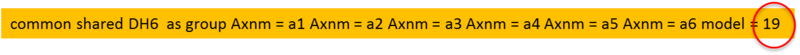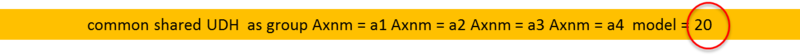# Universal DH Kinematics

## Introduction

softMC has supports many kinds of robot kinematics, several firmware coded kinematic models and one general user-defined kinematics (written by user in C-code).
All firmware coded kinematics (SCARA,DELTA, PUMA, …) assume an ideal kinematics model where angles between segments are at an exactly the predefined value (e.g. 90 deg). This is not always the case.
For example in PUAM robot kienamtics it is assumed that angle between first and second joint is exactly 90 degrees, in this model (model=2) there is no option to define different angle or an angle correction.
Only segment lengths can be manually entered. In addition to that at PUMA kinematic there exist a model constraint that all last 3 rotation axes (J4,J5,J6) must meet at same point.

For general robots:

• Up to 6DOF
• Must be Open-chain kinematics (no parallel robots)

There are two types of universal kinematics:

• PUMA “like” 6-DOF
open-chain model:Model = 19 is PUMA-like 6DOF kinematics

• General Open-Chain Kinematics
open-chain model definition:Model = 20 is general open-chain kinematics

### Difference Between Model = 20 and Model = 19

• Model 19 is specially tailored for PUMA-like robots. This means that robot need to satisfy the following conditions:
All axes are rotary.
There is exactly 6 DOF’s (axes)
Robot configuration flags (arm, elbow, wrist) are defined according most similar PUMA robot.
• Model 20 is general open-chain model, axes can be bot rotary or linear and the only limitation is that there can not be more then 6 DOF (axes).
Robot configuration flags are defined according user-defined function calls.

## Geometry Definition

Robot geometry is defined by two arrays:

1. Link [i] [j] (segment length):
1. Where i = 1-6 for segments 1 to 6
2. Where j = 1-3 for coordinates x,y,z
3. Segment length coordinates are relative to base coordinate origin placed at connection point with previous segment (without any rotation)

2. Axis [i] [j] (joint axis direction):
1. Where i = 1-6 for segments 1 to 6
2. Where j = 1-3 for coordinates x,y,z
3. Axis rotation vector relative to base coordinate origin)�In case of translational joint it is direction of the axis movement.

### User Functions

In case of general open-chain model (20) the robot configuration can not be automatically defined therefor we are using the following user functions:

• Import_c UDH_SET_MAX_CFG(byval as long,byval as long) as long Setting the max number of robot configurations (for PUMA it is 8)

• Import_c UDH_SET_CFG(byval as long, byval as long,byval as joint of xyzr) as long Setting representative robot configuration for each configuration flag.
Flag values are sett according to bitmask in same ways as in ToJoint function.

Example (SCARA):

•  ?UDH_SET_MAX_CFG(elementid,2)
•  ?UDH_SET_CFG(elementid,0,{0,-45,0,0}) ' lefty
•  ?UDH_SET_CFG(elementid,1,{0,45,0,0}) ' righty